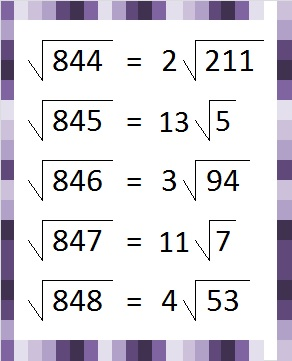# 266 Why 8 Consecutive Numbers with 6 Factors is Impossible

• 266 is a composite number.
• Prime factorization: 266 = 2 x 7 x 19
• The exponents in the prime factorization are 1, 1, and 1. Adding one to each and multiplying we get (1 + 1)(1 + 1)(1 + 1) = 2 x 2 x 2 = 8. Therefore 266 has 8 factors.
• Factors of 266: 1, 2, 7, 14, 19, 38, 133, 266
• Factor pairs: 265 = 1 x 266, 2 x 133, 7 x 38, or 14 x 19
• 266 has no square factors that allow its square root to be simplified. √266 ≈ 16.3095242, 243, 244, and 245 are the smallest four consecutive numbers that have the same number of factors. Each of them has exactly six factors, and as a result each one of their square roots can be simplified. Is it possible to have a longer string of consecutive numbers with exactly six factors? I don’t know yet. It seems reasonable that it could happen, so I am on the lookout for five, six, or seven consecutive numbers that have exactly six factors.

I won’t bother looking for a string of eight or more numbers with six factors. I already know that would be impossible. Here’s an example illustrating why:846 ruins the run because it has an additional prime factor that doubles the number of factors that 846 has in all. Further down the number line that problem might be overcome in a different set of consecutive numbers.

However, the problem with 848 is a recurring problem that will never be overcome. 848 is divisible by 8, as is every eighth number. The prime factorization of numbers that are divisible by eight must contain a power of two that is greater than or equal to three. Its number of factors calculation would have to be at least (3 + 1)(1 + 1) = 4 x 2 = 8. (The ONLY number divisible by 8 that has exactly 6 factors is 32.)

Even though the numbers from 844 to 848 don’t have the same number of factors, they still have a distinction. They are the smallest five consecutive numbers whose square roots can be simplified!1.wbhs62